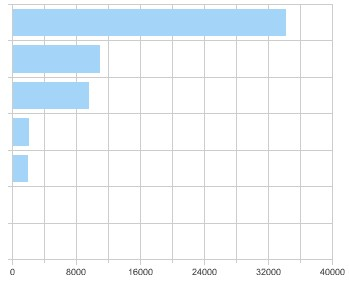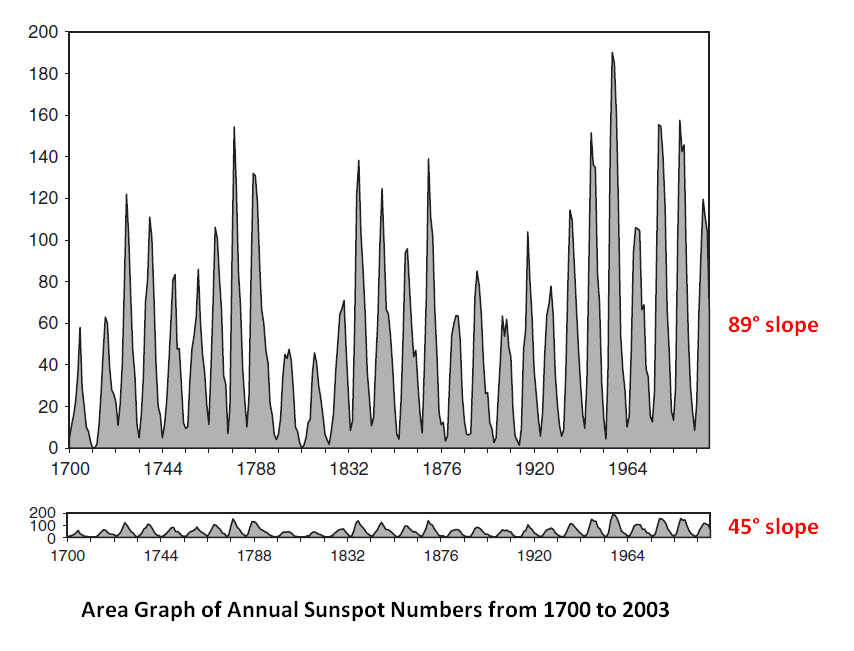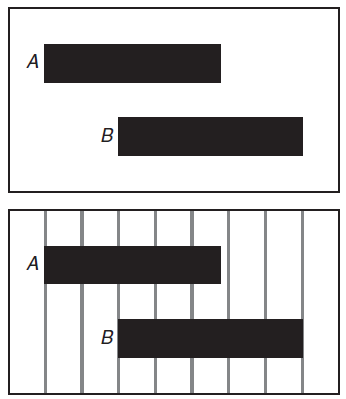# Viz - Comparison (Difference/Equality )

Comparison in Viz.

Same as Viz - (Change|Variation)

## Grid

A Reference Grid Improves the Likelihood of perceiving a difference between shapes A and B. See weber_law

## Weber Law

Ernst Heinrich Weber (1795–1878) was one of the first people to approach the study of the human response to a physical stimulus in a quantitative fashion.

Weber’s law was formulated by 19th century psychophysicist E. H. Weber.

An illustration of the Weber–Fechner law. On each side, the lower square contains 10 more dots than the upper one. However the perception is different: On the left side, the difference between upper and lower square is clearly visible. On the right side, both squares look almost the same.

See JND below

## just-noticeable difference (JND)

A just-noticeable difference or JND is the amount something must be changed in order for a difference to be noticeable, detectable at least half the time.

Also known as:

• the difference limen,
• differential threshold,
• or least perceptible difference.

The JND is a fixed proportion of the reference sensory level, and so the ratio of the JND/reference is roughly constant (that is the JND is a constant proportion/percentage of the reference level).

$$k_{jnd} = \frac{dx}{x}$$ where:

• x is the visual property of an object (example: length of a segment, number of point, light intensity)
• dx is the difference in visual property of the second object
• k is the JND constant

Discover MoreData Visualization - Bar Chart

Bar graphs plots: a categorical variable associated with quantities (number of case, sum of) histograms continuous variable associated with its bins They show: quantities as bar lengths...Text - Diff (How to compare two files ?)

One of the most that you have to perform when you work in a migration of in a concurrent development environment is to check the difference between two text files. See Diff HTML With the ins and...Viz - (Change|Variation)

Change of value for one variable. Changes are a series of visual comparison. See also: RateViz - Graph Aspect Ratio

The aspect ratio of a graph between the height and width of the axis. rate of change0963488414The Elements of Graphing Data (1994) Viewers can best understand changes in the slope of the line when...Viz - Grid

in Viz. A grid is a series of guide located at the same interval. A Reference Grid Improves the Likelihood of Perceiving a Difference Between Shapes A and B. See